﻿ Steady flow of electromagnetic fluid between parallel plates with magnetic fields - XSim

# Steady flow of electromagnetic fluid between parallel plates with magnetic fields

Update: June 1, 2017
OpenFOAM 4.x

## Case directory

\$FOAM_TUTORIALS/electromagnetics/mhdFoam/hartmann

## Summary

Calculate the steady flow of electromagnetic fluid between parallel plates with vertically applied magnetic fields (Hartmann flow).

The fluid flows in from the region inlet at 1 m/s and out from the region outlet. The magnetic field perpendicular to the flow is uniformly set with a magnetic flux density of 20 T. The calculation is performed as a two-dimensional problem with a single mesh in the Z direction.Model geometry

The magnetic field B is solved using an algorithm similar to the PISO method (B-PISO). At this time, the quantity pB, which corresponds to the pressure in the PISO method, is solved.

The meshes are as follows.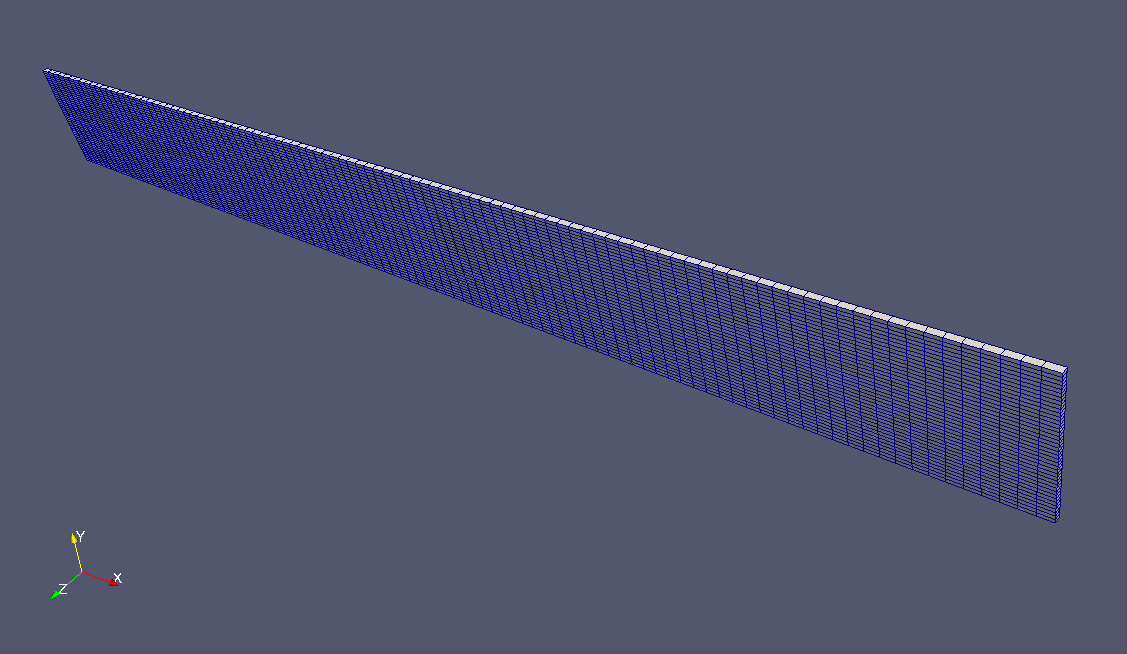Meshes

To visualize the calculated magnetic field, check "B" in the "Properties" tab on ParaView.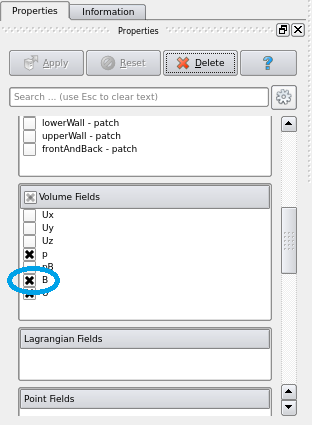Check "B"

The result is as follows.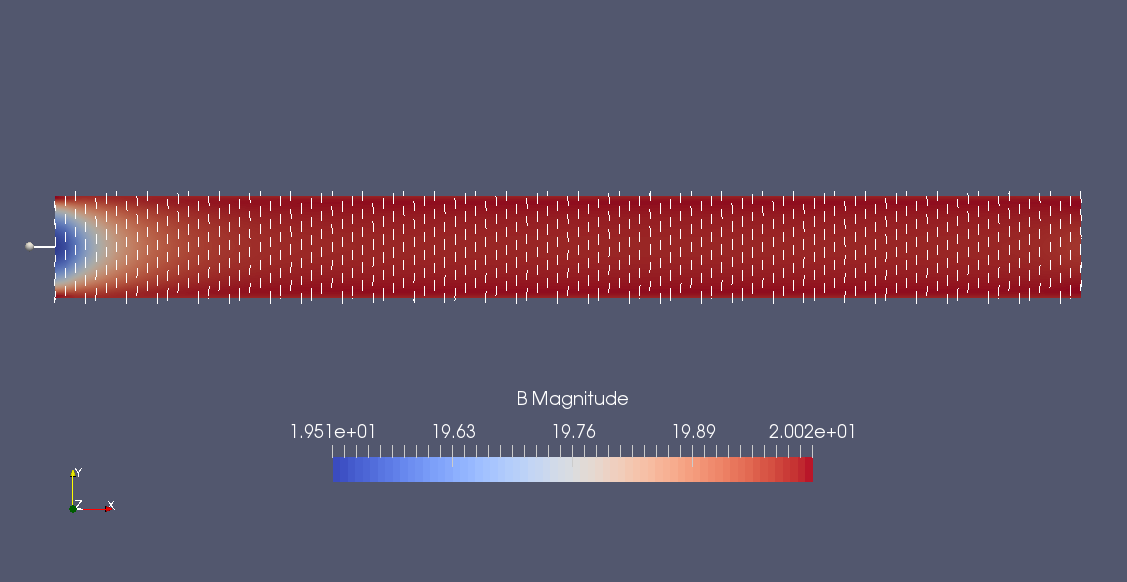Magnetic field (B)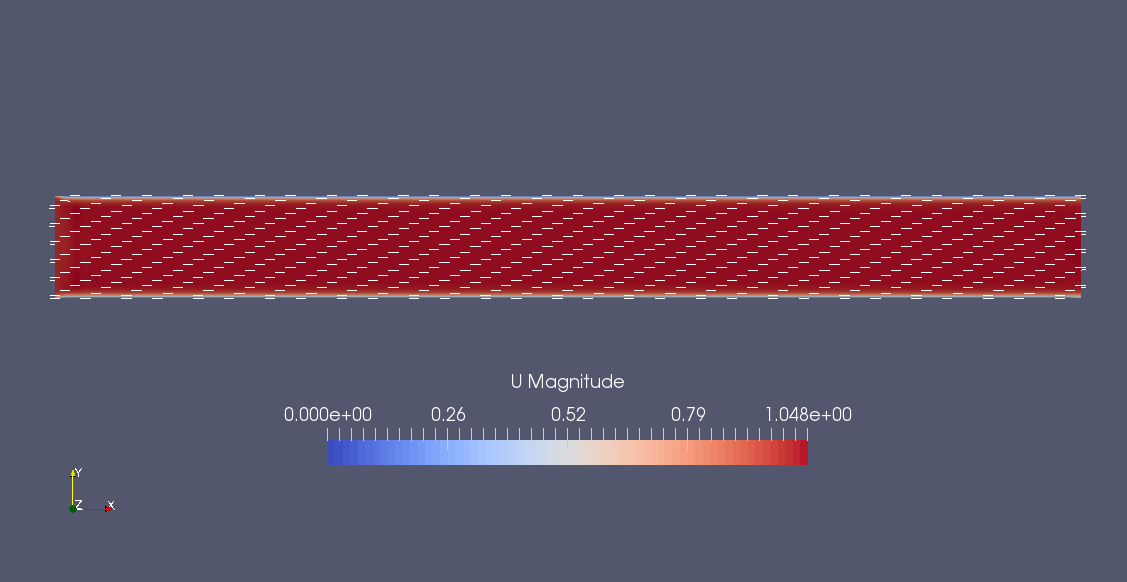Flow velocity (U)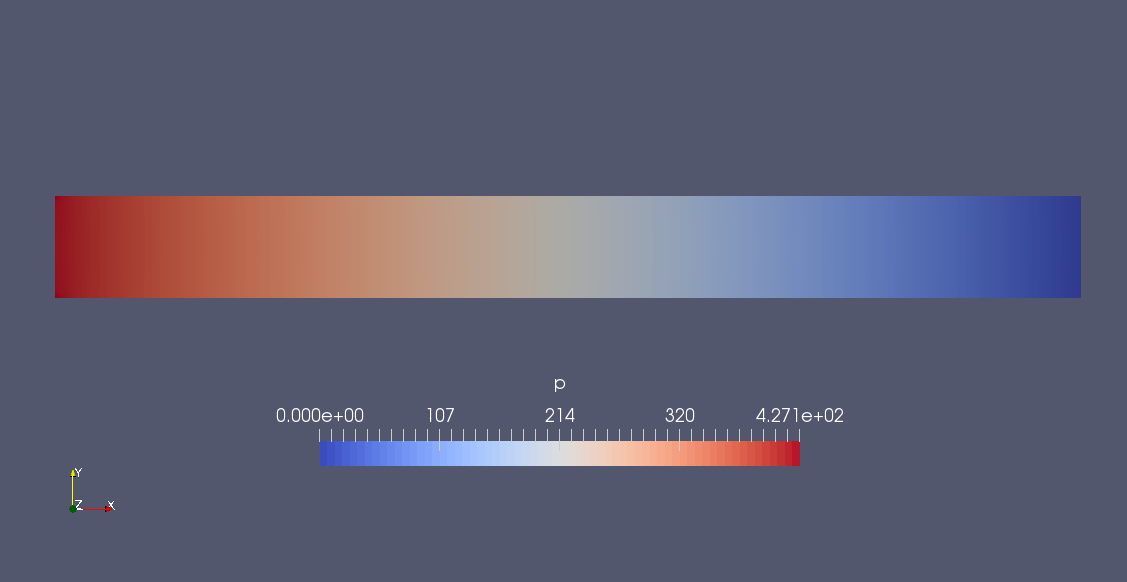Pressure (p)

We can see how the the magnetic field strength changes.

Also, when the utility postProcess is run, the X-direction component of the flow velocity is sampled on a straight line with X=10.001 according to the settings in the file system/sample.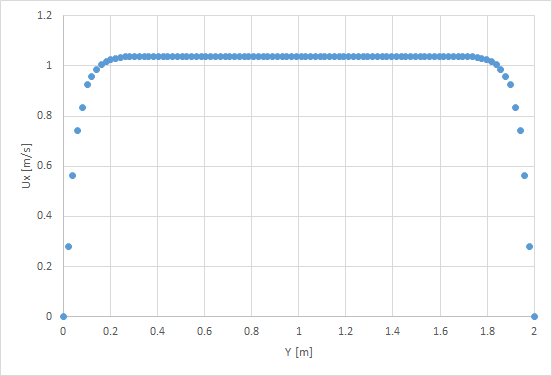The X-direction component of the flow velocity on X=10.001, at final time

Sampling results are output as a text file, which can be graphed with any spreadsheet software.

## Commands

cp -r \$FOAM_TUTORIALS/electromagnetics/mhdFoam/hartmann hartmann
cd chargedWire

blockMesh
mhdFoam
postProcess -func sample

paraFoam

Sampling is done with postProcess.

## Calculation time

--- *Single, Core(TM) i7-2600 CPU @ 3.40GHz 3.40GHz